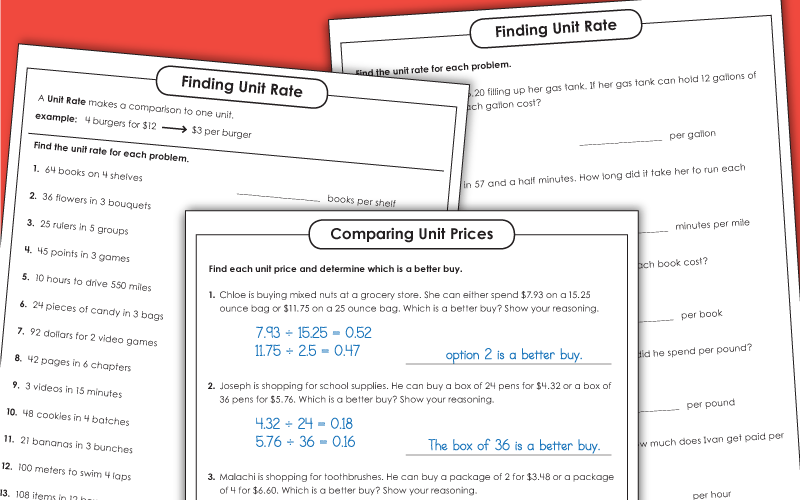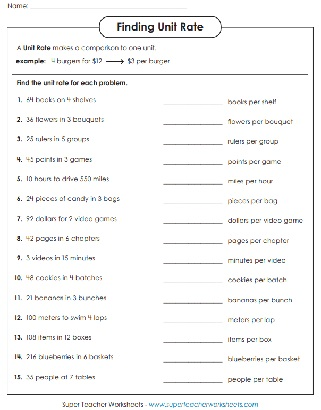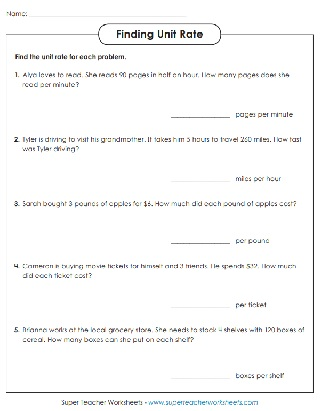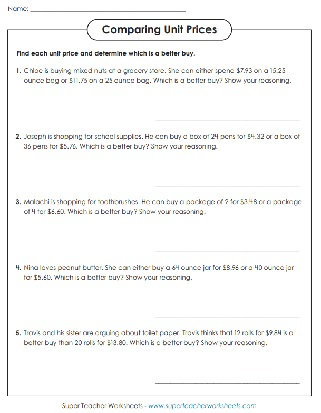# Unit Rate

On these printable worksheets, student will learn the concept of a unit rate, and they will practice solving word problems related to linear measurement, constant speed, and pricing. These math activities align closely with the following Common Core Standards: CCSS.6.RP.A.2, CCSS.6.RP.A.3.B, and CCSS.7.RP.A.1.Find the unit rate for each group. 15 questions on this page. Example: 4 burgers for \$12 -> \$3 per burger.
Read each word problem. Find the unit rate. This basic-level worksheet does not include decimals.
Determine the unit rate for each problem. This intermediate version includes decimals and fractions.
Calculate the unit price for each word problem. Then determine which is the better buy.
More Middle School Math Topics

On Super Teacher Worksheets, you'll find lots of worksheets for teaching pre-algebra, algebra, box plots, inequalities, slope, surface area, volume, and much more.

## Sample Worksheet ImagesMy Account
Site Information